CGAL 4.8.2 - Point Set Processing
User Manual

# Introduction

This CGAL component implements methods to analyze and process 3D point sets. The input is an unorganized 3D point set, possibly with normal attributes (unoriented or oriented). The input point set can be analyzed to measure geometric properties such as average spacing between the points and their k nearest neighbors. It can be processed with functions devoted to the simplification, regularization, upsampling, outlier removal, smoothing, normal estimation and normal orientation. The processing of point sets is often needed in applications dealing with measurement data, such as surface reconstruction from laser scanned data (see Figure 68.1).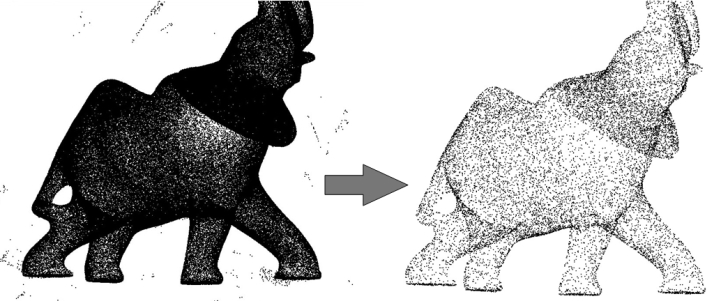Figure 68.1 Point set processing. Left: 275K points sampled on the statue of an elephant with a Minolta laser scanner. Right: point set after outlier removal, denoising and simplification to 17K points.

In the context of surface reconstruction we can position the elements of this component along the common surface reconstruction pipeline (Figure 68.2) which involves the following steps:

1. Scanning and scan alignment to produce a set of points or points with normals (alignment is not covered in CGAL);
2. Outlier removal;
3. Simplification to reduce the number of input points;
4. Smoothing to reduce noise in the input data;
5. Normal estimation and orientation when the normals are not already provided by the acquisition device; and
6. Surface reconstruction. Chapter Poisson Surface Reconstruction deals with surface reconstruction from point sets with normal attributes.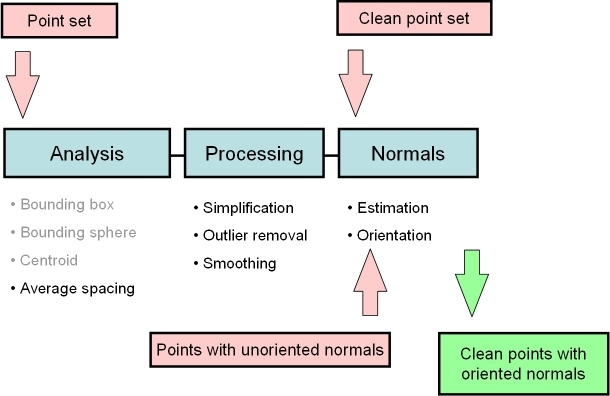Figure 68.2 Point set processing pipeline for surface reconstruction. The algorithms listed in gray are available from other CGAL components (bounding volumes and principal component analysis).

# Property Maps

The algorithms of this component take as input parameters iterator ranges of 3D points, or of 3D points with normals. The property maps are used to access the point or normal information from the input data, so as to let the user decide upon the implementation of a point with normal. The latter can be represented as, e.g., a class derived from the CGAL 3D point, or as a std::pair<Point_3<K>, Vector_3<K>>, or as a boost::tuple<..,Point_3<K>, ..., Vector_3<K> >.

The following classes described in Chapter CGAL and Boost Property Maps provide property maps for the implementations of points with normals listed above:

• Identity_property_map<T>
• First_of_pair_property_map<Pair> and Second_of_pair_property_map<Pair>
• Nth_of_tuple_property_map<N, Tuple>

Identity_property_map<Point_3> is the default value of the position property map expected by all functions in this component.

See below examples using pair and tuple property maps.

Users of this package may use other types to represent positions and normals if they implement the corresponding property maps.

Points and normals can even be stored in separate containers and accessed by their index, as any built-in vector is also a property map.

#include <CGAL/Exact_predicates_inexact_constructions_kernel.h>
#include <CGAL/grid_simplify_point_set.h>
#include <CGAL/property_map.h>
#include <vector>
#include <fstream>
// types
typedef Kernel::Point_3 Point;
typedef Kernel::Vector_3 Vector;
int main(int argc, char*argv[])
{
// Reads a .xyz point set file in points[].
std::vector<Point> points;
std::vector<Vector> normals;
const char* fname = (argc>1)?argv:"data/fin90_with_PCA_normals.xyz";
std::ifstream stream(fname);
Point p;
Vector v;
while(stream >> p >> v){
points.push_back(p);
normals.push_back(v);
}
std::cout << points.size() << " input points" << std::endl;
std::vector<std::size_t> indices(points.size());
for(std::size_t i = 0; i < points.size(); ++i){
indices[i] = i;
}
// simplification by clustering using erase-remove idiom
double cell_size = 0.05;
std::vector<std::size_t>::iterator end;
end = CGAL::grid_simplify_point_set(indices.begin(), indices.end(), &(points),cell_size);
std::size_t k = end - indices.begin();
std::cerr << "Keep " << k << " of " << indices.size() << "indices" << std::endl;
{
std::vector<Point> tmp_points(k);
std::vector<Vector> tmp_normals(k);
for(std::size_t i=0; i<k; ++i){
tmp_points[i] = points[indices[i]];
tmp_normals[i] = normals[indices[i]];
}
points.swap(tmp_points);
normals.swap(tmp_normals);
}
std::cout << points.size() << " points after the simplification" << std::endl;
return EXIT_SUCCESS;
}

# Input/Output

## Streams

We provide functions to read and write sets of points or sets of points with normals from the following ASCII file formats: XYZ (three point coordinates x y z per line or three point coordinates and three normal vector coordinates x y z nx ny nz per line), OFF (Object File Format)  and PLY (Polygon File Format).

• read_xyz_points()
• read_off_points()
• read_ply_points()
• write_off_points()
• write_xyz_points()
• write_ply_points()

Note that read_ply_points() also accepts binary PLY format in addition of ASCII.

## Example

The following example reads a point set from an input file and writes it to a file, both in the XYZ format. Positions and normals are stored in pairs and accessed through property maps.

#include <CGAL/Exact_predicates_inexact_constructions_kernel.h>
#include <CGAL/property_map.h>
#include <CGAL/IO/write_xyz_points.h>
#include <utility> // defines std::pair
#include <vector>
#include <fstream>
#include <iostream>
// types
typedef Kernel::Point_3 Point;
typedef Kernel::Vector_3 Vector;
// Point with normal vector stored as a std::pair.
typedef std::pair<Point, Vector> Pwn;
int main(int argc, char*argv[])
{
const char* fname = (argc>1)?argv:"data/oni.xyz";
// Reads a .xyz point set file in points[].
// Note: read_xyz_points_and_normals() requires an output iterator
// over points and as well as property maps to access each
// point position and normal.
std::vector<Pwn> points;
std::ifstream in(fname);
if (!in ||
in,std::back_inserter(points),
{
std::cerr << "Error: cannot read file " << fname << std::endl;
return EXIT_FAILURE;
}
// Saves point set.
// Note: write_xyz_points_and_normals() requires an output iterator
// over points as well as property maps to access each
// point position and normal.
std::ofstream out("oni_copy.xyz");
if (!out ||
out, points.begin(), points.end(),
{
return EXIT_FAILURE;
}
return EXIT_SUCCESS;
}

# Analysis

Function compute_average_spacing() computes the average spacing of all input points to their k nearest neighbor points, k being specified by the user. As it provides an order of a point set density, this function is used downstream the surface reconstruction pipeline to automatically determine some parameters such as output mesh sizing for surface reconstruction.

## Example

The following example reads a point set in the xyz format and computes the average spacing. Index, position and color are stored in a tuple and accessed through property maps.
File Point_set_processing_3/average_spacing_example.cpp

#include <CGAL/Exact_predicates_inexact_constructions_kernel.h>
#include <CGAL/compute_average_spacing.h>
#include <vector>
#include <fstream>
#include <boost/tuple/tuple.hpp>
// Types
typedef Kernel::FT FT;
typedef Kernel::Point_3 Point;
// Data type := index, followed by the point, followed by three integers that
// define the Red Green Blue color of the point.
typedef boost::tuple<int, Point, int, int, int> IndexedPointWithColorTuple;
// Concurrency
typedef CGAL::Parallel_tag Concurrency_tag;
#else
typedef CGAL::Sequential_tag Concurrency_tag;
#endif
int main(int argc, char*argv[])
{
const char* fname = (argc>1)?argv:"data/sphere_20k.xyz";
// Reads a .xyz point set file in points.
// As the point is the second element of the tuple (that is with index 1)
// we use a property map that accesses the 1st element of the tuple.
std::vector<IndexedPointWithColorTuple> points;
std::ifstream stream(fname);
if (!stream ||
stream, std::back_inserter(points),
{
std::cerr << "Error: cannot read file " << fname << std::endl;
return EXIT_FAILURE;
}
// Initialize index and RGB color fields in tuple.
// As the index and RGB color are respectively the first and third-fifth elements
// of the tuple we use a get function from the property map that accesses the 0
// and 2-4th elements of the tuple.
for(unsigned int i = 0; i < points.size(); i++)
{
points[i].get<0>() = i; // set index value of tuple to i
points[i].get<2>() = 0; // set RGB color to black
points[i].get<3>() = 0;
points[i].get<4>() = 0;
}
// Computes average spacing.
const unsigned int nb_neighbors = 6; // 1 ring
FT average_spacing = CGAL::compute_average_spacing<Concurrency_tag>(
points.begin(), points.end(),
nb_neighbors);
std::cout << "Average spacing: " << average_spacing << std::endl;
return EXIT_SUCCESS;
}

Note that other functions such as centroid or bounding volumes are found in other CGAL components:

• centroid()
• bounding_box()
• Min_sphere_of_spheres_d<Traits>

# Outlier Removal

Function remove_outliers() deletes a user-specified fraction of outliers from an input point set. More specifically, it sorts the input points in increasing order of average squared distances to their k nearest neighbors and deletes the points with largest value.

## Example

The following example reads a point set and removes 5% of the points. It uses the Identity_property_map<Point_3> property map (optional as it is the default position property map of all functions in this component.)
File Point_set_processing_3/remove_outliers_example.cpp

#include <CGAL/Exact_predicates_inexact_constructions_kernel.h>
#include <CGAL/property_map.h>
#include <CGAL/remove_outliers.h>
#include <vector>
#include <fstream>
#include <iostream>
// types
typedef Kernel::Point_3 Point;
int main(int argc, char*argv[])
{
const char* fname = (argc>1)?argv:"data/oni.xyz";
// Reads a .xyz point set file in points[].
// The Identity_property_map property map can be omitted here as it is the default value.
std::vector<Point> points;
std::ifstream stream(fname);
if (!stream ||
{
std::cerr << "Error: cannot read file " << fname << std::endl;
return EXIT_FAILURE;
}
// Removes outliers using erase-remove idiom.
// The Identity_property_map property map can be omitted here as it is the default value.
const double removed_percentage = 5.0; // percentage of points to remove
const int nb_neighbors = 24; // considers 24 nearest neighbor points
points.erase(CGAL::remove_outliers(points.begin(), points.end(),
nb_neighbors, removed_percentage),
points.end());
// Optional: after erase(), use Scott Meyer's "swap trick" to trim excess capacity
std::vector<Point>(points).swap(points);
return EXIT_SUCCESS;
}

# Simplification

Four simplification functions are devised to reduce an input point set.

Function random_simplify_point_set() randomly deletes a user-specified fraction of points from the input point set. This algorithm is fast.

Function grid_simplify_point_set() considers a regular grid covering the bounding box of the input point set, and clusters all points sharing the same cell of the grid by picking as representant one arbitrarily chosen point. This algorithm is slower than random_simplify_point_set().

Function hierarchy_simplify_point_set() provides an adaptive simplification of the point set through local clusters . The size of the clusters is either directly selected by the user or it automatically adapts to the local variation of the point set.

Function wlop_simplify_and_regularize_point_set() not only simplifies, but also regularizes downsampled points. This is an implementation of the Weighted Locally Optimal Projection (WLOP) algorithm .

## Grid Simplification Example

The following example reads a point set and simplifies it by clustering.
File Point_set_processing_3/grid_simplification_example.cpp

#include <CGAL/Exact_predicates_inexact_constructions_kernel.h>
#include <CGAL/grid_simplify_point_set.h>
#include <vector>
#include <fstream>
// types
typedef Kernel::Point_3 Point;
int main(int argc, char*argv[])
{
// Reads a .xyz point set file in points[].
std::vector<Point> points;
const char* fname = (argc>1)?argv:"data/oni.xyz";
std::ifstream stream(fname);
if (!stream ||
{
std::cerr << "Error: cannot read file " << fname << std::endl;
return EXIT_FAILURE;
}
// simplification by clustering using erase-remove idiom
double cell_size = 0.001;
points.erase(CGAL::grid_simplify_point_set(points.begin(), points.end(), cell_size),
points.end());
// Optional: after erase(), use Scott Meyer's "swap trick" to trim excess capacity
std::vector<Point>(points).swap(points);
return EXIT_SUCCESS;
}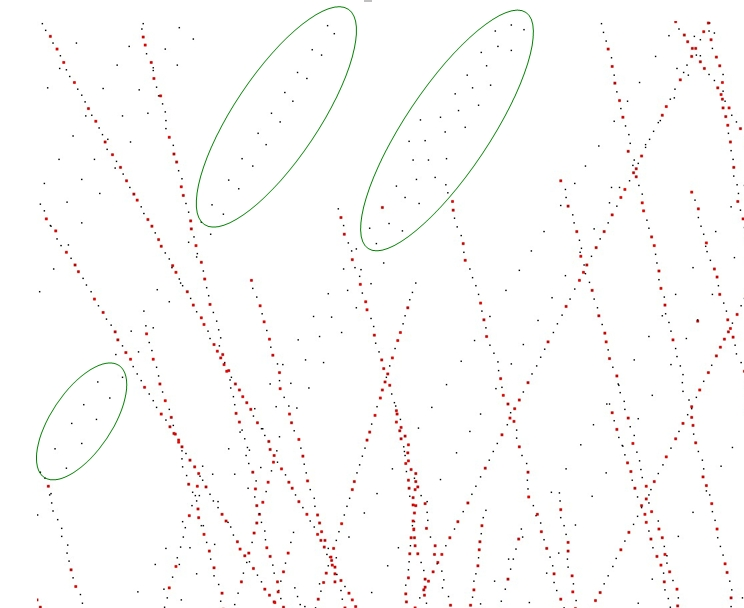Figure 68.3 Point set simplification through grid-based clustering. Removed points are depicted in red. Notice how low-density areas (in green) are not simplified.

## Hierarchy Simplification Example

The following example reads a point set and produces a set of clusters.

#include <CGAL/Exact_predicates_inexact_constructions_kernel.h>
#include <CGAL/hierarchy_simplify_point_set.h>
#include <CGAL/IO/write_xyz_points.h>
#include <CGAL/Timer.h>
#include <CGAL/Memory_sizer.h>
#include <vector>
#include <fstream>
// types
typedef Kernel::Point_3 Point;
int main(int argc, char*argv[])
{
// Reads a .xyz point set file in points[].
std::vector<Point> points;
const char* fname = (argc>1)?argv:"data/oni.xyz";
std::ifstream stream(fname);
if (!stream ||
{
std::cerr << "Error: cannot read file " << fname << std::endl;
return EXIT_FAILURE;
}
std::cout << "Read " << points.size () << " point(s)" << std::endl;
// simplification by clustering using erase-remove idiom
points.erase (CGAL::hierarchy_simplify_point_set (points.begin (), points.end (),
100, // Max cluster size
0.01), // Max surface variation
points.end ());
std::size_t memory = CGAL::Memory_sizer().virtual_size();
std::cout << points.size () << " point(s) kept, computed in "
<< task_timer.time() << " seconds, "
<< (memory>>20) << " Mib allocated." << std::endl;
std::ofstream f ("out.xyz");
CGAL::write_xyz_points (f, points.begin (), points.end ());
return EXIT_SUCCESS;
}

### Parameter: size

The hierarchy simplification algorithm recursively split the point set in two until each cluster's size is less than the parameter size.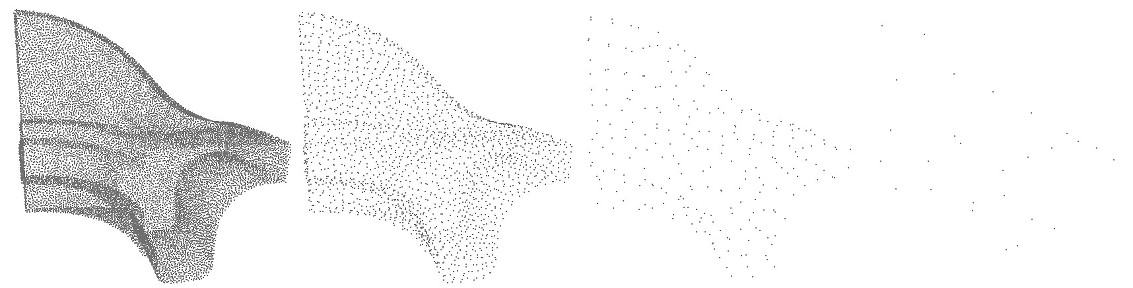Figure 68.4 Input point set and hierarchy simplification with different size parameter: $$10$$, $$100$$ and $$1000$$. In the 3 cases, var_max $$=1/3$$.

### Parameter: var_max

In addition to the size parameter, a variation parameter allows to increase simplification in monotoneous regions. For each cluster, a surface variation measure is computed using the sorted eigenvalues of the covariance matrix:

$\sigma(p) = \frac{\lambda_0}{\lambda_0 + \lambda_1 + \lambda_2}.$

This function goes from $$0$$ if the cluster is coplanar to $$1/3$$ if it is fully isotropic. If a cluster's variation is above var_max, it is splitted. If var_max is equal to $$1/3$$, this parameter has no effect and the clustering is regular on the whole point set.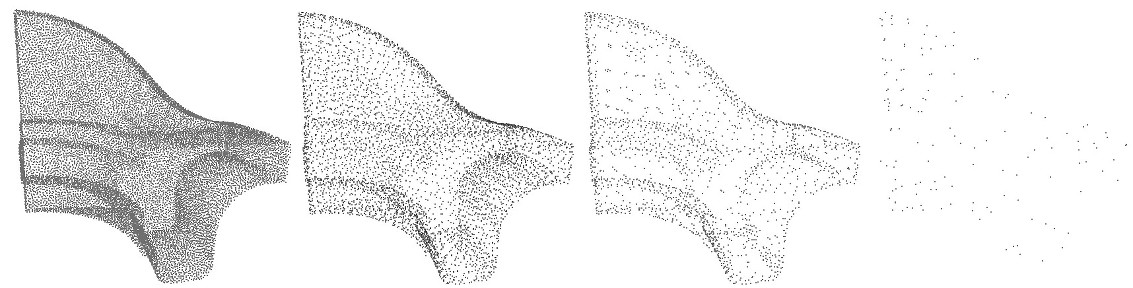Figure 68.5 Input point set and hierarchy simplification with different var_max parameter: $$0.00001$$, $$0.001$$ and $$0.1$$. In the 3 cases, size $$=1000$$.

## WLOP Simplification Example

The following example reads a point set, simplifies and regularizes it by WLOP.

#include <CGAL/Simple_cartesian.h>
#include <CGAL/wlop_simplify_and_regularize_point_set.h>
#include <CGAL/IO/write_xyz_points.h>
#include <vector>
#include <fstream>
#include <iostream>
// types
typedef Kernel::Point_3 Point;
// Concurrency
typedef CGAL::Parallel_tag Concurrency_tag;
#else
typedef CGAL::Sequential_tag Concurrency_tag;
#endif
int main(int argc, char** argv)
{
const char* input_filename = (argc>1)?argv:"data/sphere_20k.xyz";
const char* output_filename = (argc>2)?argv:"data/sphere_20k_WLOPED.xyz";
// Reads a .xyz point set file in points[]
std::vector<Point> points;
std::ifstream stream(input_filename);
if (!stream || !CGAL::read_xyz_points(stream, std::back_inserter(points)))
{
std::cerr << "Error: cannot read file " << input_filename << std::endl;
return EXIT_FAILURE;
}
std::vector<Point> output;
//parameters
const double retain_percentage = 2; // percentage of points to retain.
const double neighbor_radius = 0.5; // neighbors size.
<Concurrency_tag>
(points.begin(),
points.end(),
std::back_inserter(output),
retain_percentage,
);
std::ofstream out(output_filename);
if (!out || !CGAL::write_xyz_points(
out, output.begin(), output.end()))
{
return EXIT_FAILURE;
}
return EXIT_SUCCESS;
}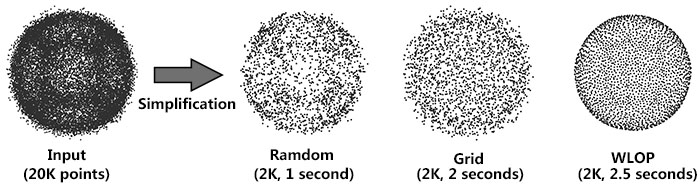Figure 68.6 Comparison for three simplification methods: Left: Random simplification result. Middle: Grid simplification result. Right: WLOP simplification result.

### Parameter: require_uniform_sampling

Computing density weights for each point is an optional preprocessing. For example, as shown in the following figure, when require_uniform_sampling is set to false, WLOP preserves the intrinsic non-uniform sampling of the original points; if require_uniform_sampling is set to true, WLOP is resilient to non-uniform sampling and generates sample points with more uniform distribution, at the expense of computational time.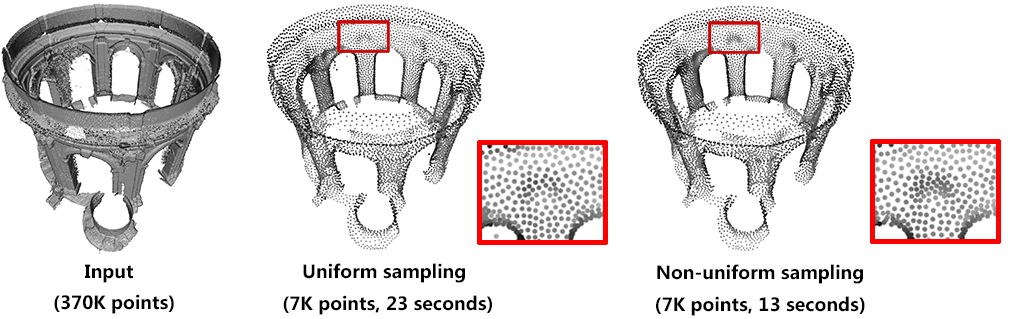Figure 68.7 Comparison between with and without density: Left: input. Middle: require_uniform_sampling = false. Right: require_uniform_sampling=true.

Usually, the neighborhood of sample points should include at least two rings of neighboring sample points. Using a small neighborhood size may not be able to generate regularized result, while using big neighborhood size will make the sample points shrink into the interior of the local surface (under-fitting). The function will use a neighborhood size estimation if this parameter value is set to default or smaller that zero.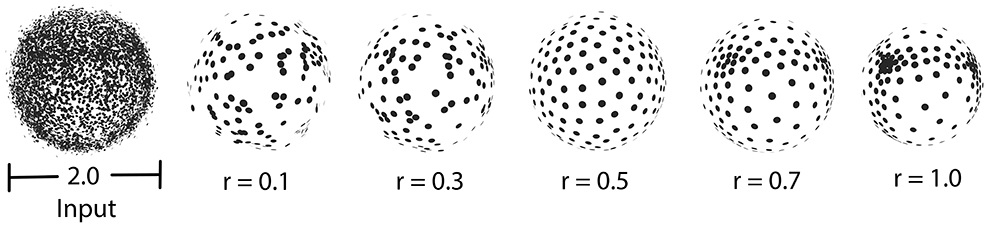Figure 68.8 Comparison between different sizes of neighbor radius.

### Parallel Performance

A parallel version of WLOP is provided and requires the executable to be linked against the Intel TBB library. To control the number of threads used, the user may use the tbb::task_scheduler_init class. See the TBB documentation for more details. We provide below a speed-up chart generated using the parallel version of the WLOP algorithm. The machine used is a PC running Windows 7 64-bits with a 4-core i7-47.nosp@m.00HQ.nosp@m.@2.40.nosp@m.GHz CPU with 8GB of RAM.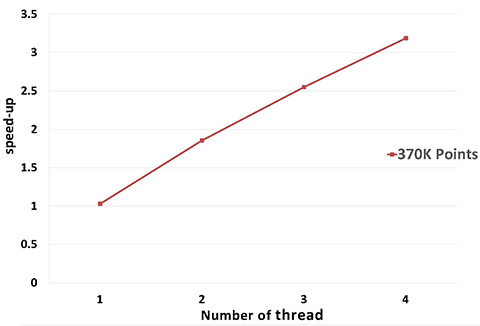Figure 68.9 Parallel WLOP speed-up, compared to the sequential version of the algorithm.

# Smoothing

Two smoothing functions are devised to smooth an input point set.

Function jet_smooth_point_set() smooths the input point set by projecting each point onto a smooth parametric surface patch (so-called jet surface) fitted over its k nearest neighbors.

Function bilateral_smooth_point_set() smooths the input point set by iteratively projecting each point onto the implicit surface patch fitted over its k nearest neighbors. Bilateral projection preserves sharp features according to the normal (gradient) information. Normals are thus required as input. For more details, see section 4 of .

## Jet Smoothing Example

The following example generates a set of 9 points close to the xy plane and smooths them using 8 nearest neighbors:
File Point_set_processing_3/jet_smoothing_example.cpp

#include <CGAL/Exact_predicates_inexact_constructions_kernel.h>
#include <CGAL/jet_smooth_point_set.h>
#include <vector>
// types
typedef Kernel::Point_3 Point;
// Concurrency
typedef CGAL::Parallel_tag Concurrency_tag;
#else
typedef CGAL::Sequential_tag Concurrency_tag;
#endif
int main(void)
{
// generate point set
std::vector<Point> points;
points.push_back(Point( 0.0, 0.0, 0.001));
points.push_back(Point(-0.1,-0.1, 0.002));
points.push_back(Point(-0.1,-0.2, 0.001));
points.push_back(Point(-0.1, 0.1, 0.002));
points.push_back(Point( 0.1,-0.1, 0.000));
points.push_back(Point( 0.1, 0.2, 0.001));
points.push_back(Point( 0.2, 0.0, 0.002));
points.push_back(Point( 0.2, 0.1, 0.000));
points.push_back(Point( 0.0,-0.1, 0.001));
// Smoothing.
const unsigned int nb_neighbors = 8; // default is 24 for real-life point sets
CGAL::jet_smooth_point_set<Concurrency_tag>(points.begin(), points.end(), nb_neighbors);
return EXIT_SUCCESS;
}

## Bilateral Smoothing Example

The following example reads a set of points with normals and smooths them via bilateral smoothing:
File Point_set_processing_3/bilateral_smooth_point_set_example.cpp

#include <CGAL/Simple_cartesian.h>
#include <CGAL/property_map.h>
#include <CGAL/IO/write_xyz_points.h>
#include <CGAL/bilateral_smooth_point_set.h>
#include <CGAL/tags.h>
#include <utility> // defines std::pair
#include <fstream>
// Types
typedef Kernel::Point_3 Point;
typedef Kernel::Vector_3 Vector;
// Point with normal vector stored in a std::pair.
typedef std::pair<Point, Vector> PointVectorPair;
// Concurrency
typedef CGAL::Parallel_tag Concurrency_tag;
#else
typedef CGAL::Sequential_tag Concurrency_tag;
#endif
int main(int argc, char*argv[])
{
const char* input_filename = (argc>1)?argv:"data/fin90_with_PCA_normals.xyz";
const char* output_filename = (argc>2)?argv:"data/fin90_with_PCA_normals_bilateral_smoothed.xyz";
// Reads a .xyz point set file in points[] * with normals *.
std::vector<PointVectorPair> points;
std::ifstream stream(input_filename);
if (!stream ||
std::back_inserter(points),
{
std::cerr << "Error: cannot read file " << input_filename << std::endl;
return EXIT_FAILURE;
}
// Algorithm parameters
int k = 120; // size of neighborhood. The bigger the smoother the result will be.
// This value should bigger than 1.
double sharpness_angle = 25; // control sharpness of the result.
// The bigger the smoother the result will be
int iter_number = 3; // number of times the projection is applied
for (int i = 0; i < iter_number; ++i)
{
/* double error = */
CGAL::bilateral_smooth_point_set <Concurrency_tag>(
points.begin(),
points.end(),
k,
sharpness_angle);
}
std::ofstream out(output_filename);
if (!out ||
out, points.begin(), points.end(),
{
return EXIT_FAILURE;
}
return EXIT_SUCCESS;
}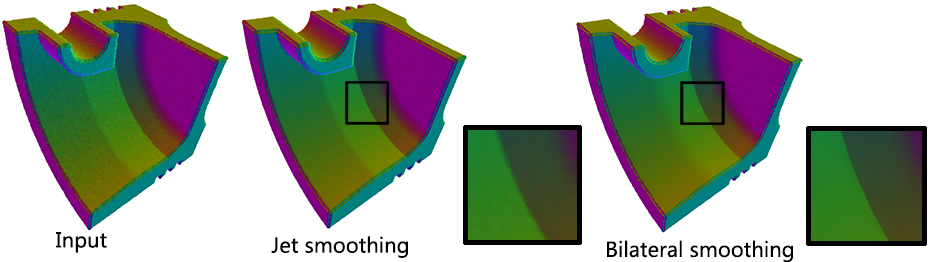Figure 68.10 Comparison for two smoothing methods: Left: Input, 250K points, normal-color mapping. Middle: Jet smoothing result, 197 seconds. Right: Bilateral smoothing result, 110 seconds.

### Parallel

Performance: A parallel version of bilateral smoothing is provided and requires the executable to be linked against the Intel TBB library. The number of threads used is controlled through the tbb::task_scheduler_init class. See the TBB documentation for more details. We provide below a speed-up chart generated using the parallel version of the bilateral smoothing algorithm. The machine used is a PC running Windows 7 64-bits with a 4-core i7-47.nosp@m.00HQ.nosp@m.@2.40.nosp@m.GHz CPU with 8GB of RAM.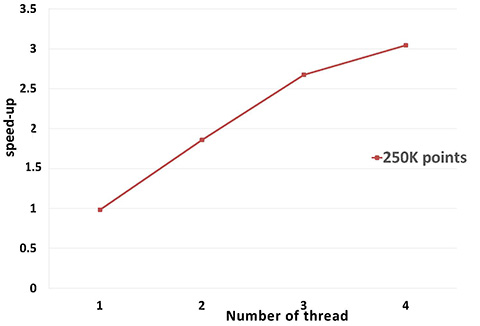Figure 68.11 Parallel bilateral smoothing speed-up, compared to the sequential version of the algorithm.

# Normal Estimation

Assuming a point set sampled over an inferred surface S, two functions provide an estimate of the normal to S at each point. The result is an unoriented normal vector for each input point.

Function jet_estimate_normals() estimates the normal direction at each point from the input set by fitting a jet surface over its k nearest neighbors. The default jet is a quadric surface. This algorithm is well suited to point sets scattered over curved surfaces.

Function pca_estimate_normals() estimates the normal direction at each point from the set by linear least squares fitting of a plane over its k nearest neighbors. This algorithm is simpler and faster than jet_estimate_normals().

Function vcm_estimate_normals() estimates the normal direction at each point from the set by using the Voronoi Covariance Measure of the point set. This algorithm is more complex and slower than the previous algorithms. It is based on the article .

# Normal Orientation

Function mst_orient_normals() orients the normals of a set of points with unoriented normals using the method described by Hoppe et al. in Surface reconstruction from unorganized points . More specifically, this method constructs a Riemannian graph over the input points (the graph of the k nearest neighbor points) and propagates a seed normal orientation within a minimum spanning tree computed over this graph. The result is an oriented normal vector for each input unoriented normal, except for the normals which cannot be successfully oriented.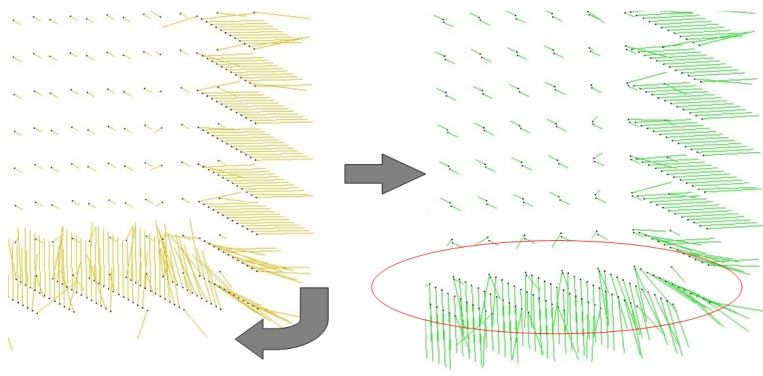Figure 68.12 Normal orientation of a sampled cube surface. Left: unoriented normals. Right: orientation of right face normals is propagated to bottom face.

## Example

The following example reads a point set from a file, estimates the normals through PCA over the 6 nearest neighbors and orients the normals:
File Point_set_processing_3/normals_example.cpp

#include <CGAL/Exact_predicates_inexact_constructions_kernel.h>
#include <CGAL/pca_estimate_normals.h>
#include <CGAL/mst_orient_normals.h>
#include <CGAL/property_map.h>
#include <utility> // defines std::pair
#include <list>
#include <fstream>
// Types
typedef Kernel::Point_3 Point;
typedef Kernel::Vector_3 Vector;
// Point with normal vector stored in a std::pair.
typedef std::pair<Point, Vector> PointVectorPair;
// Concurrency
typedef CGAL::Parallel_tag Concurrency_tag;
#else
typedef CGAL::Sequential_tag Concurrency_tag;
#endif
int main(int argc, char*argv[])
{
const char* fname = (argc>1)?argv:"data/sphere_1k.xyz";
// Reads a .xyz point set file in points[].
std::list<PointVectorPair> points;
std::ifstream stream(fname);
if (!stream ||
std::back_inserter(points),
{
std::cerr << "Error: cannot read file " << fname<< std::endl;
return EXIT_FAILURE;
}
// Estimates normals direction.
// Note: pca_estimate_normals() requires an iterator over points
// as well as property maps to access each point's position and normal.
const int nb_neighbors = 18; // K-nearest neighbors = 3 rings
CGAL::pca_estimate_normals<Concurrency_tag>(points.begin(), points.end(),
nb_neighbors);
// Orients normals.
// Note: mst_orient_normals() requires an iterator over points
// as well as property maps to access each point's position and normal.
std::list<PointVectorPair>::iterator unoriented_points_begin =
CGAL::mst_orient_normals(points.begin(), points.end(),
nb_neighbors);
// Optional: delete points with an unoriented normal
// if you plan to call a reconstruction algorithm that expects oriented normals.
points.erase(unoriented_points_begin, points.end());
return EXIT_SUCCESS;
}

# Upsampling

The function edge_aware_upsample_point_set() generates a denser point set from an input point set. This has applications in point-based rendering, hole filling, and sparse surface reconstruction. The algorithm can progressively upsample the point set while approaching the edge singularities. See  for more details.

## Example

The following example reads a point set from a file, upsamples it to get a denser result.

#include <CGAL/Simple_cartesian.h>
#include <CGAL/edge_aware_upsample_point_set.h>
#include <CGAL/IO/write_xyz_points.h>
#include <vector>
#include <fstream>
// types
typedef Kernel::Point_3 Point;
typedef Kernel::Vector_3 Vector;
// Point with normal vector stored in a std::pair.
typedef std::pair<Point, Vector> PointVectorPair;
// Concurrency
typedef CGAL::Parallel_tag Concurrency_tag;
#else
typedef CGAL::Sequential_tag Concurrency_tag;
#endif
int main(int argc, char* argv[])
{
const char* input_filename = (argc>1)?argv:"data/before_upsample.xyz";
const char* output_filename = (argc>2)?argv:"data/before_upsample_UPSAMPLED.xyz";
// Reads a .xyz point set file in points[], *with normals*.
std::vector<PointVectorPair> points;
std::ifstream stream(input_filename);
if (!stream ||
std::back_inserter(points),
{
std::cerr << "Error: cannot read file " << input_filename << std::endl;
return EXIT_FAILURE;
}
//Algorithm parameters
const double sharpness_angle = 25; // control sharpness of the result.
const double edge_sensitivity = 0; // higher values will sample more points near the edges
const double neighbor_radius = 0.25; // initial size of neighborhood.
const std::size_t number_of_output_points = points.size() * 4;
//Run algorithm
CGAL::edge_aware_upsample_point_set<Concurrency_tag>(
points.begin(),
points.end(),
std::back_inserter(points),
sharpness_angle,
edge_sensitivity,
number_of_output_points);
// Saves point set.
std::ofstream out(output_filename);
if (!out ||
out, points.begin(), points.end(),
{
return EXIT_FAILURE;
}
return EXIT_SUCCESS;
}

### Parameter: edge_sensitivity

This parameter controls where the new points are inserted. Larger values of edge-sensitivity give higher priority to inserting points along the sharp features. For example, as shown in the following figure, high value is preferable when one wants to insert more points on sharp features, where the local gradient is high, e.g., darts, cusps, creases and corners. In contrast, points are evenly inserted when edge_sensitivity is set to 0. The range of possible value is [0, 1].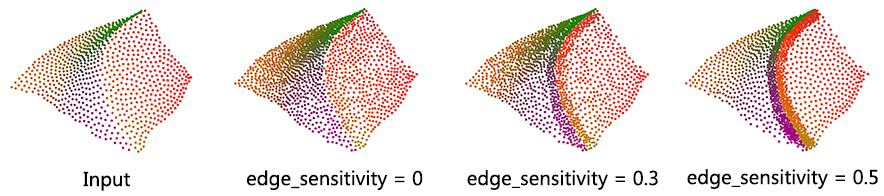Figure 68.13 Upsampling for different edge-sensitivity parameter values. The input containing 850 points is upsampled to 1,500 points in all cases depicted.

### Parameter: sharpness_angle

This parameter controls the preservation of sharp features.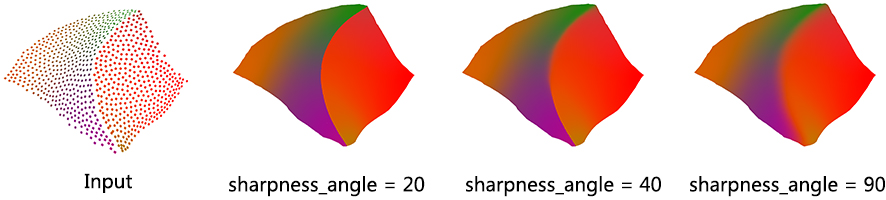Figure 68.14 Upsampling for different sharpness_angle parameter values. The input containing 850 points is upsampled to 425K points in all cases depicted.

Usually, the neighborhood of sample points should include at least one ring of neighboring sample points. Using small neighborhood size may not be able to insert new points. Using big neighborhood size can fill small holes, but points inserted on the edges could be irregular. The function will use a neigbhorhood size estimation if this parameter value is set to default or smaller that zero.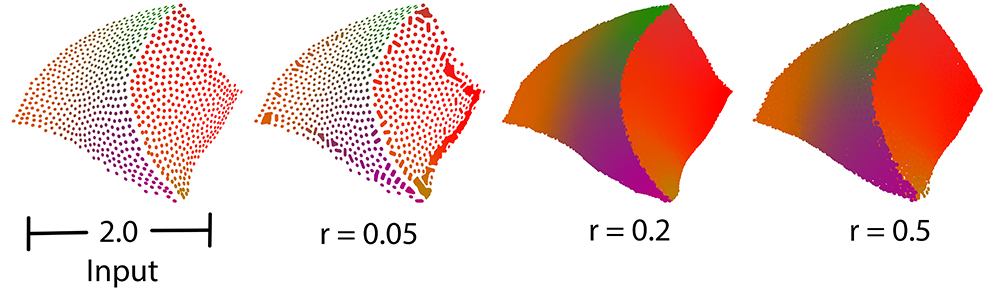Figure 68.15 Comparison between different sizes of neighbor radius.

# Feature Edges Estimation

Function vcm_is_on_feature_edge() indicates if a points belong to a feature edges of the point set using its Voronoi Covariance Measure. It is based on the article .

It first computes the VCM of the points set using compute_vcm(). Then, it estimates which points belong to a sharp edge by testing if a ratio of eigenvalues is greater than a given threshold.

## Example

The following example reads a point set from a file, estimates the points that are on sharp edges:
File Point_set_processing_3/edges_example.cpp

#include <CGAL/Exact_predicates_inexact_constructions_kernel.h>
#include <CGAL/vcm_estimate_edges.h>
#include <CGAL/property_map.h>
#include <utility> // defines std::pair
#include <vector>
#include <fstream>
#include <boost/foreach.hpp>
// Types
typedef Kernel::Point_3 Point;
typedef Kernel::Vector_3 Vector;
// Point with normal vector stored in a std::pair.
typedef std::pair<Point, Vector> PointVectorPair;
typedef std::vector<PointVectorPair> PointList;
typedef CGAL::cpp11::array<double,6> Covariance;
int main (int , char**) {
// Reads a .xyz point set file in points[].
std::list<PointVectorPair> points;
std::ifstream stream("data/fandisk.off");
if (!stream ||
std::back_inserter(points),
{
std::cerr << "Error: cannot read file data/fandisk.off" << std::endl;
return EXIT_FAILURE;
}
// Estimates covariance matrices per points.
double R = 0.2,
r = 0.1;
std::vector<Covariance> cov;
CGAL::compute_vcm(points.begin(), points.end(), point_pmap, cov, R, r, Kernel());
// Find the points on the edges.
// Note that this step is not expensive and can be done several time to get better results
double threshold = 0.16;
std::ofstream output("points_on_edges.xyz");
int i = 0;
BOOST_FOREACH(const PointVectorPair& p, points)
{
if (CGAL::vcm_is_on_feature_edge(cov[i], threshold))
output << p.first << "\n";
++i;
}
return 0;
}

# Implementation History

Pierre Alliez and Laurent Saboret contributed the initial component. Nader Salman contributed the grid simplification. Started from GSoC'2013, three new algorithms were implemented by Shihao Wu and Clément Jamin: WLOP, bilateral smoothing and upsampling. Started from GSoC'2014, Jocelyn Meyron with the help of Quentin Mérigot introduced the computation of the Voronoi covarience measure of a point set, as well as the normal and feature edge estimation functions based on it.